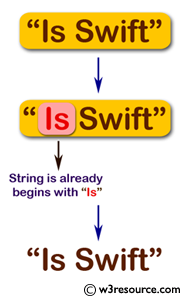﻿ Swift Basic Programming Exercise: Add "Is" to the front of a given string with condition - w3resource# Swift Basic Programming Exercise: Add "Is" to the front of a given string with condition

## Swift Basic Programming: Exercise-5 with Solution

Write a Swift program to add "Is" to the front of a given string. However, if the string already begins with "Is", return the given string.

Pictorial Presentation:Sample Solution:

Swift Code:

``````import Foundation
func isstring(word: String) -> String {
if word.hasPrefix("Is") == true
{
return word
}
else
{
return "Is \(word)"
}
}

print(isstring(word: "Is Swift"))
print(isstring(word: "Swift"))
```
```

Sample Output:

```Is Swift
Is Swift
```

Swift Programming Code Editor:

Improve this sample solution and post your code through Disqus

What is the difficulty level of this exercise?

﻿